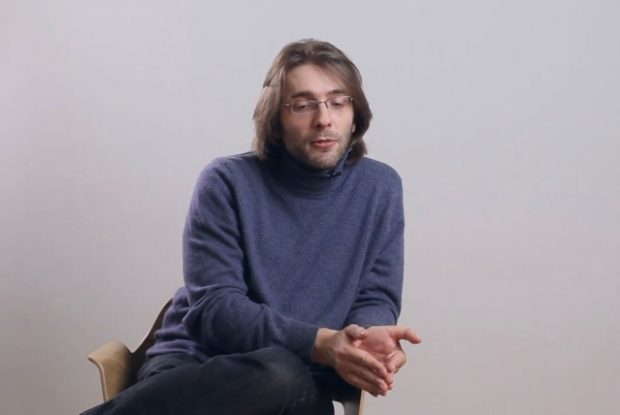Formal logic

Philosopher Dennis Bonnay on metamathematics, set theory, and the Gödel’s theorem

videos | December 2, 2016

What is the relationship between axioms and the real world? Why may proofs be more important for mathematics than the truth? How did computer science develop? These and other questions are answered by Assistant Professor in the Department of Philosophy, Paris Ouest University, Dennis Bonnay.

Formal logic which is the way in that mathematicians reflect upon what they do and try to describe their practice in mathematical ways. Sometimes formal logic is called metamathematics precisely because it is a systematic study of mathematics by mathematical means.Philosopher Denis Bonnay on Kant's "Critique of Pure Reason", logical inferences, and attempts to divide mathematics and logic

You could say that the central concept of mathematics is the notion of computation, of an algorithm, and so there is another branch of formal logic, which is a systematic study of algorithms. This is sort of a borderline between formal logic and the foundations of computer science, because indeed the foundations of computer science first developed as a branch of formal logic.

One of the things people nowadays are interested in is to try to unify mathematics, so to try to find a way to describe in a systematic manner all kinds of mathematical objects and structures that mathematicians use and all of the kinds of reasoning that we use.Assistant Professor, Department of Philosophy, Paris Ouest University
Did you like it? Share it with your friends!
Published items
0779
To be published soon
+92

Most viewed

New